# JP5490828B2 - Linear system coefficient estimation method, integrated circuit using the same, touch panel system, and electronic device - Google Patents

Linear system coefficient estimation method, integrated circuit using the same, touch panel system, and electronic device Download PDF

## Info

Publication number
JP5490828B2
JP5490828B2 JP2012002600A JP2012002600A JP5490828B2 JP 5490828 B2 JP5490828 B2 JP 5490828B2 JP 2012002600 A JP2012002600 A JP 2012002600A JP 2012002600 A JP2012002600 A JP 2012002600A JP 5490828 B2 JP5490828 B2 JP 5490828B2
Authority
JP
Japan
Prior art keywords
unit
linear sum
sum signal
noise
touch panel
Prior art date
Legal status (The legal status is an assumption and is not a legal conclusion. Google has not performed a legal analysis and makes no representation as to the accuracy of the status listed.)
Active
Application number
JP2012002600A
Other languages
Japanese (ja)
Other versions
JP2013142993A (en
Inventor

Original Assignee
シャープ株式会社
Priority date (The priority date is an assumption and is not a legal conclusion. Google has not performed a legal analysis and makes no representation as to the accuracy of the date listed.)
Filing date
Publication date
Application filed by シャープ株式会社 filed Critical シャープ株式会社
Priority to JP2012002600A priority Critical patent/JP5490828B2/en
Publication of JP2013142993A publication Critical patent/JP2013142993A/en
Application granted granted Critical
Publication of JP5490828B2 publication Critical patent/JP5490828B2/en
Application status is Active legal-status Critical
Anticipated expiration legal-status Critical

## Images

•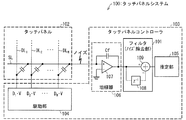•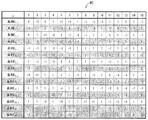•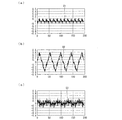•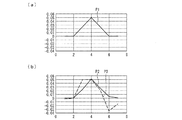•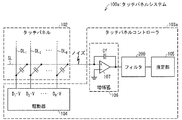•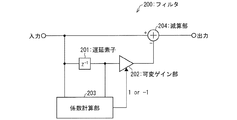•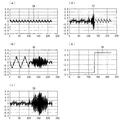•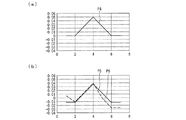•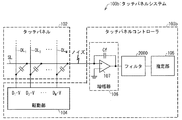•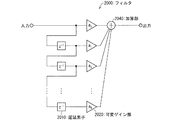•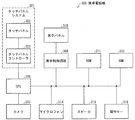••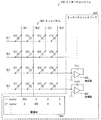•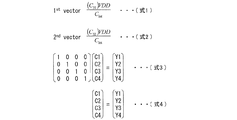## Classifications

• GPHYSICS
• G06COMPUTING; CALCULATING; COUNTING
• G06FELECTRIC DIGITAL DATA PROCESSING
• G06F3/00Input arrangements for transferring data to be processed into a form capable of being handled by the computer; Output arrangements for transferring data from processing unit to output unit, e.g. interface arrangements
• G06F3/01Input arrangements or combined input and output arrangements for interaction between user and computer
• G06F3/03Arrangements for converting the position or the displacement of a member into a coded form
• G06F3/041Digitisers, e.g. for touch screens or touch pads, characterised by the transducing means
• G06F3/044Digitisers, e.g. for touch screens or touch pads, characterised by the transducing means by capacitive means
• GPHYSICS
• G06COMPUTING; CALCULATING; COUNTING
• G06FELECTRIC DIGITAL DATA PROCESSING
• G06F3/00Input arrangements for transferring data to be processed into a form capable of being handled by the computer; Output arrangements for transferring data from processing unit to output unit, e.g. interface arrangements
• G06F3/01Input arrangements or combined input and output arrangements for interaction between user and computer
• G06F3/03Arrangements for converting the position or the displacement of a member into a coded form
• G06F3/041Digitisers, e.g. for touch screens or touch pads, characterised by the transducing means
• G06F3/0416Control or interface arrangements specially adapted for digitisers
• G06F3/0418Control or interface arrangements specially adapted for digitisers for error correction or compensation, e.g. based on parallax, calibration or alignment

## Description

The present invention relates to a linear system coefficient estimation method for estimating or detecting capacitance values of capacitances configured in a matrix, an integrated circuit using the same, a touch panel system, and an electronic apparatus.

Capacitance detection circuit for detecting a distribution of capacitance values of a capacitance matrix formed between M drive lines and L sense lines as a device for detecting capacitance values distributed in a matrix However, this is disclosed in Patent Document 1. In the capacitance detection device, when the touch panel is touched with a finger or a pen, the capacitance value of the capacitance becomes small at the touched position. By detecting the change in the capacitance value, The contact position with the touch panel by the pen is detected.

FIG. 13 is a schematic diagram showing a configuration of a conventional touch panel system 900. FIG. 14 is a diagram for explaining a driving method of the touch panel system 900. The touch panel system 900 includes a touch panel 902 and a touch panel controller 903. Touch panel 902 has drive lines DL1 to DL4, sense lines SL1 to SL4, and capacitances C11 to C44 arranged at positions where drive lines DL1 to DL4 and sense lines SL1 to SL4 intersect. . The touch panel controller 903 includes a drive unit 904 and an amplifier 908.

The following describes how the touch panel system 900 converts the capacitance value into a measurement value (detection value) by using a method.

The drive unit 904 drives the drive lines DL1 to DL4 based on the 4 × 4 code sequence shown in Equation 3 of FIG. If the code sequence element is “1”, the drive unit 904 applies the power supply voltage VDD, and if the element is “0”, it applies zero volts.

The touch panel system 900 includes four amplifiers 908 arranged so as to correspond to the sense lines SL1 to SL4. The four amplifiers 908 receive and amplify the linear sums Y1 to Y4 of the capacitances along the sense lines SL1 to SL4 driven by the driving unit 904.

Specifically, for example, in the first drive among the four times of the 4 rows × 4 columns code sequence, the drive unit 904 applies the power supply voltage VDD to the drive line DL1, and the remaining drive lines DL2 to DL2 are driven. Apply zero volts to DL4. Then, for example, from the sense line SL3, an output corresponding to the capacitance C31 represented by Expression 1 in FIG. 14 is supplied to the amplifier 908 as the measured value Y1.

In the second drive, the power supply voltage VDD is applied to the drive line DL2, and zero volts is applied to the remaining drive lines DL1, DL3, and DL4. Then, an output corresponding to the capacitance C32 represented by the expression 2 in FIG. 14 is supplied from the sense line SL3 to the amplifier 908 as the measured value Y2.

Similarly, in the third drive, the power supply voltage VDD is applied to the drive line DL3, and zero volts is applied to the remaining drive lines. Thereafter, in the fourth drive, the power supply voltage VDD is applied to the drive line DL4, and zero volts is applied to the remaining drive lines. The measurement values Y3 and Y4 corresponding to the capacitances C3 and C4 are obtained by the third driving and the fourth driving, respectively.

As described above, the measured values Y1, Y2, Y3, and Y4 are associated with the capacitance values C1, C2, C3, and C4 as shown in Expression 3 and Expression 4 in FIG.

JP 2005-152223 A (published June 16, 2005)

As described above, the touch panel 900 can obtain a measured value (detected value) from the capacitance value.

However, the touch panel system 900 can acquire only capacitance data intersecting with one drive line in one measurement, the noise component is not reduced, and it is difficult to correctly detect the capacitance change of the capacitance. There's a problem.

That is, the conventional touch panel system 900 has a problem that it is easily affected by noise, in other words, has low noise resistance.

The present invention has been made in view of the above problems, and an object thereof is to provide a linear system coefficient estimation method having high noise resistance, an integrated circuit using the same, a touch panel system, and an electronic apparatus. .

The capacitance value estimation method according to the present invention is based on M (N is a plurality) M dimensions of M capacitances existing at the intersections of a touch line sense line and M (M is a plurality) drive lines. A drive unit that drives in parallel based on a vector, a linear sum signal acquisition unit that receives N linear sum signals based on the charges of the capacitance, and a noise removal unit that removes noise from the N linear sum signals And a touch panel system including an integrated circuit having an estimation unit that estimates the M capacitance values by an inner product operation of a signal based on the N linear sum signals and the N M-dimensional vectors, a capacitance value estimation method for estimating the M capacitance value, the noise removal unit, superimposed on the linear sum signal before Symbol N linear sum signal obtained by the acquisition unit noise Filter the linear sum signal A noise removal step of removing by grayed, the estimation unit, and a signal based on the N linear sum signal removed of noise by said noise removing step, the inner product operation of the N M-dimensional vector An estimation step of estimating the M capacitance values.

According to the above feature, noise superimposed on the N linear sum signals of the target coefficient is removed by filtering the linear sum signal, and the signal based on the N linear sum signals from which the noise has been removed; The values of the M target coefficients are estimated by an inner product operation with the N M-dimensional vectors.

Therefore, the values of M target coefficients are estimated after noise is removed by filtering of the linear sum signal. Accordingly, the estimated values of the M target coefficients are not affected by noise. That is, the linear system coefficient estimation method according to the present invention is a linear system coefficient estimation method with high noise tolerance.

In the electrostatic capacitance value estimation method according to the present invention, the noise removing unit includes a discrete-time filter and a subtraction unit and a delay unit, Oite the noise removing step, (i) the delay unit The linear sum signal from the linear sum signal acquisition unit is delayed and output, and (ii) the subtraction unit is output from the delay unit from the linear sum signal from the linear sum signal acquisition unit It is preferable to subtract the sum signal .

According to the said structure, the filter used in a noise removal process is a discrete time filter.

Therefore, a filter used for filtering can be easily configured with one or more delay elements and an adder as main components.

In the capacitance value estimation method according to the present invention, the delay unit is configured to delay the linear sum signal obtained from the linear sum signal acquisition unit by a predetermined number of clocks. K code sequences (K <N) are selected from N code sequences based on a long N M sequence so that a code sequence corresponding to a predetermined number of clocks delayed by the delay unit is not included. Preferably, the N M-dimensional vectors are configured.

When N M-dimensional vectors are configured using an M-sequence that is easily implemented in hardware, a vector D2 obtained by delaying a certain M-dimensional vector D1 by a delay clock is also included in the N M-dimensional vectors. It is included in N M-dimensional vectors. When the target coefficient is estimated by calculation from the linear sum signal including both D1 and D2, the calculation may be complicated due to the above-described relationship between D1 and D2.

However, according to the above configuration, a code sequence obtained by delaying a certain code sequence by a delay clock is included in K M-dimensional vectors selected from N code sequences based on an M sequence having a code length of N. Is not included.

Therefore, the calculation for estimating the target coefficient is not complicated.

The capacitance value estimation method according to the present invention, the noise removing unit includes an adaptive filter that includes a subtraction unit and the delay elements and the coefficient calculation unit and the variable gain section, Oite the noise removing step, (i) The delay element outputs an output signal obtained by delaying the linear sum signal obtained from the linear sum signal acquisition unit, and (ii) the coefficient calculation unit is obtained by the linear sum signal acquisition unit. A correlation between the N linear sum signals and the output signal from the delay element; and (iii) the variable gain unit is configured to output the delay element according to the correlation calculation result by the coefficient calculation unit. (Iv) The subtraction unit subtracts the output signal given gain by the variable gain unit from the linear sum signal from the linear sum signal acquisition unit. It is preferable.

According to the above configuration, the adaptive filter self-adapts the transfer function to the input linear sum signal.

For this reason, even if the noise superimposed on the input linear sum signal changes, it is filtered by the optimum filter for the changed noise. Therefore, noise can be removed optimally.

Alternatively, according to the above configuration, it is possible to select a transfer function that can attenuate the noise most effectively even when the characteristics of the noise change, and thus provide a linear system coefficient estimation method with high noise resistance. Can do.

The integrated circuit according to the present invention is based on N (N is a plurality) M-dimensional vectors of M capacitances existing at the intersections of the touch line sense lines and M (M is a plurality) drive lines. a driving unit for driving in parallel, the an integrated circuit that includes a linear sum signal acquisition unit for receiving the N linear sum signal based on the electric charge of the capacitance, before obtained by the linear sum signal acquisition unit the noise superimposed on the serial N linear sum signal, and a noise removing unit for removing by filtering the linear sum signal, a signal based on the N linear sum signal removed of noise by the pre-Symbol noise removing unit And an estimation unit for estimating the M electrostatic capacitance values by an inner product operation with the N M-dimensional vectors.

According to the above feature, the noise removing unit removes the noise superimposed on the N linear sum signals of the target coefficient by filtering the linear sum signal, and the estimating unit removes the N pieces of noise from which the noise has been removed. The values of the M target coefficients are estimated by an inner product operation of a signal based on a linear sum signal and the N M-dimensional vectors.

Therefore, the values of the M target coefficients are estimated after removing the noise of the linear sum signal. Accordingly, the estimated values of the M target coefficients are not affected by noise. That is, the integrated circuit according to the present invention is an integrated circuit having high noise resistance.

In the integrated circuit according to the present invention, the noise removing unit includes: (i) a delay unit that delays and outputs the linear sum signal from the linear sum signal acquisition unit; and (ii) an output from the linear sum signal acquisition unit. It is preferable to include a discrete time filter including a subtracting unit that subtracts the linear sum signal output from the delay unit from the linear sum signal .

According to the above configuration, the noise removing unit can be easily configured mainly using one or a plurality of delay elements and an adder.

An integrated circuit according to the present invention, the delay unit, the linear sum signal the linear sum signal obtained from the obtaining unit, delays a predetermined number of clock, the drive unit is based on the M sequence code length N K code sequences (K <N) are selected from the N code sequences so as not to include a code sequence corresponding to a predetermined number of delay clocks delayed by the delay unit , and the N M sequences are selected. It is preferable to construct a dimension vector .

According to the above configuration, the K M-dimensional vectors selected from the N code sequences based on the M sequences of the code length N by the M-dimensional vector configuration unit are delayed by a delay clock with respect to a certain code sequence. Does not include the coded code sequence.

Therefore, the calculation for estimating the target coefficient is not complicated.

In the integrated circuit according to the present invention, the noise removal unit includes: (i) a delay element that outputs an output signal obtained by delaying the linear sum signal obtained from the linear sum signal acquisition unit; and (ii) the linear sum. A coefficient calculation unit for calculating a correlation between the N linear sum signals obtained by the signal acquisition unit and the output signal from the delay element; and (iii) according to the calculation result of the correlation by the coefficient calculation unit. A variable gain unit that gives a gain to the output signal from the delay element; and (iv) the output signal that is gained by the variable gain unit from the linear sum signal from the linear sum signal acquisition unit. It is preferable to include an adaptive filter including a subtracting unit that subtracts .

According to the above configuration, the adaptive filter included in the noise removal unit self-adapts the transfer function to the input linear sum signal.

Therefore, even if the noise superimposed on the input linear sum signal changes, the noise removing unit performs filtering using a filter that is optimal for the changed noise. Therefore, noise can be removed optimally.

A touch panel system according to the present invention includes the integrated circuit according to the present invention, and the touch panel having the sense lines and the M drive lines, and the integrated circuit includes the M drive lines and the sense lines. The M capacitance values of the capacitance at the intersection with are estimated .

According to the above feature, the noise removing unit provided in the integrated circuit included in the touch panel system of the present invention filters the noise that is superimposed on the N linear sum signals of the target coefficient, by filtering the linear sum signal. The estimation unit estimates the value of the M target coefficients by an inner product operation of the signal based on the N linear sum signals from which noise is removed and the N M-dimensional vectors.

Therefore, the values of the M target coefficients are estimated after removing the noise of the linear sum signal. Accordingly, the estimated values of the M target coefficients are not affected by noise. That is, the touch panel system according to the present invention is a touch panel system with high noise resistance.

An electronic apparatus according to the present invention includes an integrated circuit according to the present invention, the touch panel having the sense line and the M drive lines, and a display panel that is superimposed on the touch panel or has the touch panel built therein. And the integrated circuit estimates M capacitance values of the capacitance at the intersection of the M drive lines and the sense lines .

According to the above feature, the noise removal unit provided in the integrated circuit provided in the electronic apparatus of the present invention filters the linear sum signal for noise superimposed on the N linear sum signals of the target coefficient. The estimation unit estimates the value of the M target coefficients by an inner product operation of the signal based on the N linear sum signals from which noise is removed and the N M-dimensional vectors.

Therefore, the values of the M target coefficients are estimated after removing the noise of the linear sum signal. Accordingly, the estimated values of the M target coefficients are not affected by noise. That is, the electronic device according to the present invention is an electronic device with high noise resistance.

As described above, the capacitance value estimation method according to the present invention uses N (N is N) capacitances existing at the intersections of the touch line sense lines and M drive lines (M is a plurality). A plurality of drive units that are driven in parallel based on M-dimensional vectors, a linear sum signal acquisition unit that receives N linear sum signals based on charges of the capacitance, and noise of the N linear sum signals. An integrated circuit having a noise removing unit for removing, and an estimating unit for estimating the M capacitance values by an inner product operation of a signal based on the N linear sum signals and the N M-dimensional vectors. in a touch panel system, said a capacitance value estimation method for estimating the M capacitance value, the noise removal unit, the linear sum signal acquisition SL before obtained by the unit N linear sum signal The noise superimposed on A noise removal step of removing by filtering, the estimation unit comprises a signal based on the N linear sum signal noise has been removed by said noise removing step, the inner product operation between the N M-dimensional vector And an estimation step of estimating the M capacitance values.

Thereby, there is an effect that it is possible to provide a linear system coefficient estimation method having high noise tolerance.

It is a schematic diagram which shows the structure of the touchscreen system of this invention. It is a figure which shows the specific example of the M series whose code length is 15. (A) is a figure which shows the linear sum signal before noise application, (b) is a figure which shows the signal after noise application, (c) is a figure which shows the output signal from a filter. (A) is a figure which shows the linear sum signal before noise application, (b) is a figure which shows the signal after noise application in which the characteristic changed, (c) is a noise application using the filter which is not an adaptive filter. It is a figure which shows the signal at the time of processing a later signal. It is a schematic diagram which shows the structure of the touchscreen system of this invention provided with the filter. It is a schematic diagram which shows the structure of the filter used for the touchscreen system of this invention. (A) is a figure which shows the linear sum signal before the noise application in which the characteristic changed, (b) is a figure which shows the signal after the noise application in which the characteristic changed, (c) is a coefficient cannot be changed. It is a figure which shows the signal at the time of processing the signal after the said noise application using a noise filter. (D) is a figure which shows the signal at the time of processing the signal after the said noise application using a filter, (e) is a figure which shows the output signal of a coefficient calculation part. (A) is a figure which shows the capacity | capacitance pattern input into the filter which can change a coefficient, (b) is a figure which shows the capacity | capacitance pattern estimated by the filter from the capacity | capacitance pattern. It is a schematic diagram which shows the structure of the touchscreen system of this invention provided with the other filter which can change a coefficient. It is a schematic diagram which shows the structure of the filter which can change the coefficient used for the touchscreen system of this invention. It is a functional block diagram which shows the structure of the mobile telephone which concerns on an example of the electronic device of this invention. It is a schematic diagram which shows the structure of the touchscreen system which concerns on this invention. It is a schematic diagram which shows the structure of the conventional touch panel system. It is a figure which shows the structure of the code series for driving a touch panel system.

Hereinafter, embodiments of the present invention will be described in detail with reference to FIGS. It should be noted that the dimensions, materials, shapes, relative arrangements, etc. of the components described in each embodiment are not intended to limit the scope of the present invention only, unless otherwise specified, but only for explanation. Not too much.
(Configuration that is the premise of the embodiment)
(Example of linear system coefficient estimation method; touch panel system 800)
FIG. 12 is a schematic diagram showing a configuration of a touch panel system 800 according to a reference example of the present invention.

The touch panel system 800 includes a touch panel 802 and a touch panel controller 803. The touch panel 802 has M drive lines DL1 to DLM and a plurality of sense lines. However, in order to simplify the description, it is assumed that only one sense line SL is provided as the sense line. The position where the driveline DL1~DLM and sense lines SL intersect, the capacitance value of C 1 -C M each capacitance C1~CM is disposed.

The touch panel controller 803 is provided with a drive unit 804. The driving unit 804 drives the drive lines DL1 to DLM in parallel. If the element of the code sequence H is “1”, the driving unit 804 applies the voltage V, and if the element is “−1”, the voltage −V is applied.

The touch panel controller 803 has an amplifier 806 arranged at a position corresponding to the sense line SL. The amplifier 806 receives and amplifies the linear sum signal S j represented by the following (formula 5) along the capacitance sense line SL driven by the drive unit 804.

Here, N M-dimensional vectors are denoted as Di, j . The target coefficient A k to be estimated is calculated by the inner product calculation.

It is expressed. Substituting the aforementioned S j into Equation 6,

Given in. If the vectors D i, j (i = 1,..., M; j = 1,... N) are orthogonal to each other, the inner product of the same vectors is 1, and the inner product of different vectors is Because it becomes 0

It becomes. However, k = 1,..., M.

Now, when noise No j is superimposed on the sense line, the linear sum S j is

It becomes. The target coefficient A k estimated by the inner product calculation is

Alternatively, depending on the orthogonal condition of the vector D i, j described above,

It becomes.

A method of estimating and determining the target coefficient by linear calculation in this way is called a linear system coefficient estimation method.

In the linear system coefficient estimation method of the touch panel system 800, an error corresponding to the inner product of the noise No j and the vector D i, j is an estimated value of the target coefficient Ak (the second term on the right side of (Expression 11)), as in the above expression. ), The target coefficient A k is easily affected by noise. In other words, the target coefficient A k determined by the linear system coefficient estimation method has low noise resistance. Therefore, the touch panel system 800 has low noise resistance.
(Embodiment 1)
(Configuration of touch panel system 100)
A touch panel system 100 according to the present invention is shown in FIG.

The touch panel system 100 includes a touch panel 102 and a touch panel controller 103. The touch panel 102 has M drive lines DL1 to DLM and one sense line SL. The position where the driveline DL1~DLM and sense lines SL intersect, the capacitance value of C 1 -C M each capacitance C1~CM is disposed.

The touch panel controller 103 is provided with a drive unit 104. The drive unit 104 drives the drive lines DL1 to DLM in parallel. If the element of the code sequence H is “1”, the drive unit 104 applies the voltage V, and if the element is “−1”, the drive unit 104 applies the voltage −V.

The touch panel controller 103 has an amplifier 106 disposed at a position corresponding to the sense line SL. The amplifier 106 includes an operational amplifier 107 and an integration capacitor Cf having one end connected to the non-inverting input terminal of the operational amplifier 107 and the other end connected to the output of the operational amplifier 107. The amplifier 106 receives and amplifies the linear sum signal along the capacitance sense line SL driven by the driving unit 104.

The touch panel system 100 has the same configuration as the touch panel system 800 according to the reference illustrated in FIG. The difference between the touch panel system 100 and the touch panel system 800 is that the touch panel system 100 includes a filter 101 (noise removal unit, discrete time filter) between the amplifier 106 and the estimation unit 105. 101 is not included. In this embodiment, the number of sense lines SL is 1 (SL), and the number of drive lines DL is 7 (DL1 to DL7).

The filter 101 delays and supplies the linear sum signal from the amplifier 106, and subtracts the linear sum signal output from the delay device 108 from the linear sum signal from the amplifier 106 and supplies it to the estimation unit 105. And a subtractor 109.

The estimation unit 105 estimates M capacitance values by an inner product operation of a signal based on the linear sum signal from which noise has been removed by the filter 101 and N M-dimensional vectors.
(Operation example of touch panel system 100)
In the present embodiment, the sense line capacitance is driven using an M-sequence having a code length of 15. FIG. 2 shows an example of an M-sequence code sequence MC having a code length of 15. In this embodiment, since the number of drive lines is 7, seven of the 15 sequences E1, j to E15, j shown in FIG. 2 are used. The filter 101 of this embodiment includes a block (delay unit 108) that delays the signal by one clock. Therefore, for example, when the sequence E1, j in FIG. 2 is used, a signal obtained by delaying the sequence E2, j by one clock becomes the sequence E1, j, but it should be avoided to use these sequences simultaneously. Therefore, in the present embodiment, a series Di, j that is Di, j = E2i-1, j (i = 1,..., 7) is used.

Thus, the 15 7-dimensional vectors according to Embodiment 1 do not include a code sequence that is delayed by a delay clock from 15 code sequences based on an M-sequence code sequence MC having a code length of 15. 7 code sequences (K <N) are selected and configured.

FIG. 3A shows the linear sum signal S1 before the noise is superimposed, FIG. 3B shows the signal S2 after the noise is superimposed, and FIG. 3C shows the output signal S3 of the filter 101. The vertical axis represents normalized signal amplitude, and the horizontal axis represents time. It can be seen that the amplitude of the noise of the output signal S3 is lower than that of the signal S2 after the noise is superimposed.

Next, FIG. 4A shows the capacitance pattern P1 formed on the touch panel 102, and FIG. 4B shows the capacitance pattern P2 estimated by the estimation unit 105. The broken line is the capacitance pattern P3 when the filter 101 of the present invention is not used, and the solid line is the estimated capacitance pattern P2 when the filter 101 of the present invention is used. The vertical axis indicates the normalized capacity value, and the horizontal axis indicates the drive line number where the capacity is installed. The capacity pattern P2 using the filter 101 is an estimated pattern closer to the input capacity pattern P1 than the capacity pattern P3 not using the filter 101.

In the touch panel system 100, instead of the filter 101, a filter whose transfer function can be changed with respect to the linear sum signal may be used. Then, the touch panel system 100 with high noise resistance can be provided by selecting a transfer function that can attenuate noise most effectively.
(Linear system coefficient estimation method of the present invention)
An example of the linear system coefficient estimation method according to the present invention used in the touch panel system 100 will be described.

In the touch panel system 100, noise is attenuated by performing filtering on N linear sum signals of the target coefficients obtained by driving a plurality of target coefficients in parallel using N M-dimensional vectors. The transfer function of the filter 101 used in the filtering and Hn = 1-z -1.

Linear sum signal with capacitance value C i (i = 1,..., M)

On the other hand, when the filter 101 is applied,

It becomes. In (Equation 13), when the frequency of the noise signal is small, No j −No j−1 ≈0, which indicates that the noise can be reduced.

However, the second term on the right side of the above equation includes Di , j-1 . When an M sequence that is easily implemented in hardware is used as the M-dimensional vector D i, j , a vector obtained by delaying a certain vector by one clock becomes another vector. Here, D i, j−1 = D i + 1, j can be expressed. By substituting this into the second term on the right side of (Equation 13), So j becomes

It becomes. At this time, if the noise is almost zero, the target coefficient A k estimated by the inner product calculation is expressed by (Expression 6) and (Expression 14):

It can be expressed. Here, if the inner product of different vectors is negligibly small,

It becomes. In this case, the target coefficient A k is a difference between adjacent coefficients C k and C k−1 . there,

C k can be estimated by calculating the coefficient B k .

In addition, it is possible to estimate C k while avoiding the use of a vector obtained by delaying a certain vector by one clock. For example, if an M sequence E i, j that is 2N M-dimensional vectors is prepared and defined by E 2i, j = D i, j ,

Is

It becomes.

Therefore, the target coefficient A k estimated by the inner product operation is calculated from (Equation 2) and (Equation 19) when the noise is almost zero.

It becomes. From the definition of D i, j = E 2i, j , D i, j does not include (different) E 2i + 1 , so if the inner product of different vectors is negligibly small,

It becomes.

Thus, according to the linear system coefficient estimating method of this embodiment, the formula representing the target coefficients A k to be estimated does not include the noise No j. Therefore, the target coefficient A k is not affected by the noise No j .

Therefore, according to the linear system coefficient estimation method of the present embodiment, it is possible to provide a touch panel system 100 that can reduce noise and has high noise resistance.
(Embodiment 2)
As another example of the filter used in the integrated circuit, the touch panel system, and the electronic apparatus of the present invention, a filter 200 and a filter 2000 (adaptive filter) whose coefficients can be changed will be described with reference to FIGS.

First, an example of a signal when a filter used in an integrated circuit, a touch panel system, and an electronic device is changed from a characteristic having noise characteristics to a characteristic different from that when the coefficient cannot be changed is shown in FIG. ), As shown in FIG. FIG. 7A shows the linear sum signal S4 before applying noise whose characteristics have changed, and FIG. 7B shows the signal S5 after applying noise whose characteristics have changed. FIG. 7C shows a signal S6 obtained by processing the signal S5 using a noise filter whose coefficients cannot be changed. As can be seen from the figure, after the noise characteristics change, the noise filter cannot attenuate the noise.
In FIG. 5, the schematic diagram of a structure of the touchscreen system 100a of this invention provided with the filter 200 is shown. A difference from the touch panel system 100 described above is a touch panel controller 103 a including a filter 200 different from the filter 100. FIG. 6 shows a schematic diagram of the configuration of the filter 200. The filter 200 includes a delay element 201, a variable gain unit 202, a coefficient calculation unit 203, and a subtraction unit 204 that subtracts the signal from the variable gain unit 202 from the input signal. Here, the coefficient calculation unit 203 calculates the correlation between the input signal of the filter 200 and the output signal of the delay element 201, and outputs 1 if the correlation is positive, and outputs -1 if the correlation is negative. The variable gain unit 202 has a gain of 1 when the output signal of the coefficient calculation unit 203 is 1 with respect to the output signal of the delay element 201 and -1 when the output signal is -1.

A signal S7 obtained by filtering the signal S5 shown in FIG. 7B using the filter 200 is shown in FIG. 7D. Further, an output signal S8 from the coefficient calculation unit 203 is shown in FIG. According to the figure, it can be seen that the noise can be attenuated by the filter 200 even after the noise characteristics have changed.

Next, FIG. 8A shows a capacitance pattern P4 formed on the touch panel 102, and FIG. 8B shows an estimation by the estimation unit 105 when the capacitance pattern P4 is input to the touch panel controller 103a. The capacitance pattern P5 is shown. In FIG. 8B, a broken line is an estimated capacitance pattern P6 when the filter of the present invention is not used, and a solid line is an estimated capacitance pattern P5 when the filter 200 is used. The vertical axis indicates the normalized capacity value, and the horizontal axis indicates the drive line number where the capacity is installed. By using the filter, an estimated point pattern P5 closer to the capacitance pattern P4 formed on the touch panel 102 was obtained.

As described above, by using the filter 200 whose coefficient can be changed, the noise can be attenuated even when the noise characteristic changes.
FIG. 9 is a schematic diagram illustrating a configuration of a touch panel system 100b according to the present embodiment including a touch panel controller 103b including a filter 2000. FIG. 10 shows a filter 2000 as an example of a filter whose coefficient can be changed, which is different from the filter 200. The same reference numerals are given to the same components as those described above. Therefore, detailed description of these components will not be repeated.

Although FIGS. 1 and 6 show an example of a filter having one delay element for one clock, the filter 2000 has a structure using a plurality of delay elements 2010.

The filter 2000 is an FIR (Finite Impulse Response) filter including N delay elements 2010 and an adder 2040. In the filter 2000, the signal obtained by delaying the input signal by i (i = 0,..., N) clocks is weighted by the weight a i in the variable gain unit 2020, and then added by the adding unit 2040 and output. . By adaptively changing the weight a i , a transfer function that can attenuate the noise most effectively can be selected even when the characteristics of the noise change, and a device with high noise resistance can be provided.

In the second embodiment, the filter coefficient is 1 or -1, but other values may be used. In this embodiment, the filter coefficient is determined by signal correlation calculation, but may be determined using another method such as the LMS method.
(Embodiment 3)
A cellular phone 300 will be described with reference to FIG. 11 as an example of an electronic device equipped with a touch panel controlled by the integrated circuit of the present invention.

FIG. 11 is a functional block diagram showing the configuration of the mobile phone 300 according to the present embodiment. The cellular phone 300 includes a CPU 310, a RAM 312, a ROM 311, a camera 313, a microphone 314, a speaker 315, an operation key 316, a display panel 318, a display control circuit 309, and a touch panel system 301. . Each component is connected to each other by a data bus.

CPU 310 controls the operation of mobile phone 300. CPU 310 executes a program stored in ROM 311, for example. The operation key 316 receives an instruction input from the user to the mobile phone 300. The RAM 312 volatilely stores data generated by execution of a program by the CPU 310 or data input through the operation keys 316. The ROM 311 stores data in a nonvolatile manner.

The ROM 311 is a ROM capable of writing and erasing, such as an EPROM (Erasable Programmable Read-Only Memory) or a flash memory. Although not shown in FIG. 11, the mobile phone 300 may include an interface (IF) for connecting to another electronic device by wire.

The camera 313 captures a subject according to the operation of the operation key 316 by the user. The image data of the photographed subject is stored in the RAM 312 or an external memory (for example, a memory card). The microphone 314 receives user's voice input. The mobile phone 300 digitizes the input voice (analog data). Then, the mobile phone 300 sends the digitized voice to a communication partner (for example, another mobile phone). The speaker 315 outputs sound based on, for example, music data stored in the RAM 312.

The touch panel system 301 includes a touch panel 302 and a touch panel controller 303. CPU 310 controls the operation of touch panel system 301. For example, the CPU 310 executes a program stored in the ROM 311. The RAM 312 volatilely stores data generated by the CPU 310 executing the program. The ROM 311 stores data in a nonvolatile manner.

The display panel 318 displays images stored in the ROM 311 and the RAM 312 by the display control circuit 309. The display panel 318 is superimposed on the touch panel 302 or contains the touch panel 302.

The present invention is not limited to the above-described embodiments, and various modifications are possible within the scope of the claims, and other embodiments obtained by appropriately combining the technical means disclosed in the above-described embodiments. The form is also included in the technical scope of the present invention.

INDUSTRIAL APPLICABILITY The present invention can be used for a linear system coefficient estimation method that estimates or detects capacitance values of capacitances configured in a matrix, and an integrated circuit, a touch panel system, and an electronic device using the same.

100, 301 Touch panel system 101 Filter (noise removal unit, discrete time filter)
103, 303 Touch panel controller (integrated circuit)
104 Driver 105 Estimator 106 Amplifier 107 Operational Amplifier
108 delay unit (delay unit)
109 Subtractor (subtraction unit)
200 filter (noise removal unit, adaptive filter)
201 Delay Element 202 Variable Gain Unit 203 Coefficient Calculation Unit 300 Mobile Phone (Electronic Device)
302 Touch panel 309 Display control circuit 310 CPU
311 ROM
312 RAM
313 Camera 314 Microphone 315 Speaker 316 Operation key 318 Display panel
806 amplifier (linear sum signal acquisition unit)
2000 filter (noise removal unit, discrete time filter, adaptive filter)
Code sequence by MCM sequence S1-S8 signal P1-P3 capacity pattern

## Claims (10)

1. A drive unit that drives M capacitances existing at the intersections of the sense line of the touch panel and M drive lines (M is a plurality) in parallel based on N (N is a plurality) M-dimensional vectors; A linear sum signal acquisition unit that receives N linear sum signals based on the charge of the capacitance, a noise removal unit that removes noise of the N linear sum signals, and a signal based on the N linear sum signals wherein the N number of the touch panel system with an integrated circuit having an estimation unit for the inner product operation of the M-dimensional vector estimates the M capacitance value, the static to estimate the M capacitance value A method for estimating a capacitance value ,
Wherein the noise removal unit, the noise superimposed on the previous SL N linear sum signal obtained by the linear sum signal acquiring unit, a noise removal step of removing by filtering the linear sum signal,
The estimation unit estimates the M capacitance values by an inner product operation of a signal based on the N linear sum signals from which noise has been removed by the noise removal step and the N M-dimensional vectors. A capacitance value estimation method comprising: an estimation step.
2. The noise removing unit includes a discrete time filter including a delay unit and a subtraction unit,
Oite the noise removing step, (i) the delay unit, said from linear sum signal acquisition unit linear sum signal is delayed output, (ii) the subtraction unit, from the linear sum signal acquisition unit The capacitance value estimation method according to claim 1 , wherein a linear sum signal output from the delay unit is subtracted from the linear sum signal .
3. The delay unit is configured to delay the linear sum signal obtained from the linear sum signal acquisition unit by a predetermined number of clocks,
The driving unit includes K code sequences (K <) so that a code sequence corresponding to a predetermined number of clocks delayed by the delay unit is not included from N code sequences based on an M sequence having a code length of N. 3. The method of estimating a capacitance value according to claim 2 , wherein N) is selected to constitute the N M-dimensional vectors .
4. The noise removing unit includes an adaptive filter including a delay element, a coefficient calculating unit, a variable gain unit, and a subtracting unit,
Oite the noise removing step, (i) the delay element, the linear sum outputs an output signal obtained by delaying the linear sum signal obtained from the signal acquisition unit, (ii) the coefficient calculator, the A correlation between the N linear sum signals obtained by the linear sum signal acquisition unit and the output signal from the delay element is calculated; and (iii) the variable gain unit calculates the correlation by the coefficient calculation unit. According to the result, a gain is given to the output signal from the delay element, and (iv) the subtractor gives a gain from the linear sum signal from the linear sum signal acquisition unit by the variable gain unit. 2. The capacitance value estimation method according to claim 1 , wherein the output signal is subtracted .
5. A drive unit that drives M capacitances existing at the intersections of the sense line of the touch panel and M drive lines (M is a plurality) in parallel based on N (N is a plurality) M-dimensional vectors; An integrated circuit comprising a linear sum signal acquisition unit for receiving N linear sum signals based on the charge of the capacitance ,
A noise removing unit that removes by the noise superimposed on the previous SL N linear sum signal obtained by the linear sum signal acquisition unit, for filtering the linear sum signal,
A signal based on the N linear sum signal removed of noise by the pre-Symbol noise removing unit, and a estimation unit that estimates the M capacitance value by the inner product operation between the N M-dimensional vector further integrated circuit characterized by comprising.
6. The noise removing unit includes: (i) a delay unit that delays and outputs the linear sum signal from the linear sum signal acquisition unit; and (ii) the delay from the linear sum signal from the linear sum signal acquisition unit. 6. The integrated circuit according to claim 5, further comprising: a discrete time filter including a subtracting unit that subtracts the linear sum signal output from the unit .
7. The delay unit delays the linear sum signal obtained from the linear sum signal acquisition unit by a predetermined number of clocks,
The driving unit includes K code sequences (K) such that a code sequence corresponding to a predetermined number of delay clocks delayed by the delay unit is not included from N code sequences based on an M sequence having a code length of N. 7. The integrated circuit according to claim 6 , wherein <N) is selected to constitute the N M-dimensional vectors .
8. The noise removing unit includes: (i) a delay element that outputs an output signal obtained by delaying the linear sum signal obtained from the linear sum signal acquisition unit; and (ii) the linear sum signal acquisition unit. A coefficient calculation unit for calculating a correlation between the N linear sum signals and the output signal from the delay element; and (iii) the output from the delay element in accordance with a calculation result of the correlation by the coefficient calculation unit. A variable gain unit that gives a gain to the signal; and (iv) a subtracting unit that subtracts the output signal given gain by the variable gain unit from the linear sum signal from the linear sum signal acquisition unit. 6. The integrated circuit according to claim 5, further comprising an adaptive filter provided .
9. An integrated circuit according to any one of claims 5 to 8,
The touch panel having the sense line and the M drive lines ;
The touch panel system , wherein the integrated circuit estimates M capacitance values of the capacitance at an intersection of the M drive lines and the sense lines .
10. An integrated circuit according to any one of claims 5 to 8,
The touch panel having the sense line and the M drive lines ;
It is overlaid on the touch panel or comprises a display panel incorporating the touch panel ,
The electronic apparatus is characterized in that the integrated circuit estimates M capacitance values of the capacitance at the intersection of the M drive lines and the sense lines .
JP2012002600A 2012-01-10 2012-01-10 Linear system coefficient estimation method, integrated circuit using the same, touch panel system, and electronic device Active JP5490828B2 (en)

## Priority Applications (1)

Application Number Priority Date Filing Date Title
JP2012002600A JP5490828B2 (en) 2012-01-10 2012-01-10 Linear system coefficient estimation method, integrated circuit using the same, touch panel system, and electronic device

## Applications Claiming Priority (4)

Application Number Priority Date Filing Date Title
JP2012002600A JP5490828B2 (en) 2012-01-10 2012-01-10 Linear system coefficient estimation method, integrated circuit using the same, touch panel system, and electronic device
CN201380004869.7A CN104094201A (en) 2012-01-10 2013-01-09 Linear system coefficient estimation method, integrated circuit employing same, touch panel system, and electronic apparatus
PCT/JP2013/050246 WO2013105584A1 (en) 2012-01-10 2013-01-09 Linear system coefficient estimation method, integrated circuit employing same, touch panel system, and electronic apparatus
US14/370,889 US9189118B2 (en) 2012-01-10 2013-01-09 Linear system coefficient estimation method, integrated circuit employing same, touch panel system, and electronic apparatus

## Publications (2)

Publication Number Publication Date
JP2013142993A JP2013142993A (en) 2013-07-22
JP5490828B2 true JP5490828B2 (en) 2014-05-14

# Family

## Family Applications (1)

Application Number Title Priority Date Filing Date
JP2012002600A Active JP5490828B2 (en) 2012-01-10 2012-01-10 Linear system coefficient estimation method, integrated circuit using the same, touch panel system, and electronic device

## Country Status (4)

US (1) US9189118B2 (en)
JP (1) JP5490828B2 (en)
CN (1) CN104094201A (en)
WO (1) WO2013105584A1 (en)

## Families Citing this family (5)

* Cited by examiner, † Cited by third party
Publication number Priority date Publication date Assignee Title
JP5973072B2 (en) * 2013-06-20 2016-08-23 シャープ株式会社 Touch panel controller, integrated circuit, touch panel device, and electronic device
JP2015035053A (en) * 2013-08-08 2015-02-19 デクセリアルズ株式会社 Electrostatic capacitance type touch panel
CN106575183B (en) 2014-08-25 2020-02-14 3M创新有限公司 Capacitance-based touch device with reduced interference
JP6410699B2 (en) * 2015-10-14 2018-10-24 アルプス電気株式会社 Input device, input device control method, and program for causing computer to execute input device control method
US9740356B1 (en) * 2016-02-05 2017-08-22 Pixart Imaging Inc. Capacitive touch system using differential sensing and operating method thereof

## Family Cites Families (14)

* Cited by examiner, † Cited by third party
Publication number Priority date Publication date Assignee Title
JPH0477929B2 (en) * 1987-06-04 1992-12-09 Sony Tektronix Corp
JP3434415B2 (en) * 1996-07-05 2003-08-11 アルプス電気株式会社 Coordinate input device
JP4364609B2 (en) * 2003-11-25 2009-11-18 アルプス電気株式会社 Capacitance detection circuit and fingerprint sensor using the same
WO2005091677A1 (en) * 2004-03-22 2005-09-29 Toa Corporation Multi-channel system identification device
JP4453710B2 (en) * 2007-03-19 2010-04-21 セイコーエプソン株式会社 Liquid crystal device, electronic apparatus and position detection method
WO2009107415A1 (en) * 2008-02-27 2009-09-03 セイコーインスツル株式会社 Proximity detector and proximity detection method
TWI420374B (en) * 2008-09-08 2013-12-21 Innolux Corp Sensing circuit for capacitive touch panel and electronic device using the same
US8605037B2 (en) * 2008-10-21 2013-12-10 Atmel Corporation Noise reduction in capacitive touch sensors
EP2491478A4 (en) * 2009-10-20 2014-07-23 Cypress Semiconductor Corp Method and apparatus for reducing coupled noise influence in touch screen controllers.
JP5361688B2 (en) 2009-12-04 2013-12-04 Ｕｄトラックス株式会社 Exhaust purification device
JP5295090B2 (en) * 2009-12-18 2013-09-18 株式会社ワコム Indicator detection device
CN102156594B (en) * 2010-02-12 2013-08-21 联咏科技股份有限公司 Touch-sensing system, capacitance sensing circuit and capacitance sensing method
US9244569B2 (en) * 2010-03-31 2016-01-26 Stmicroelectronics Asia Pacific Pte Ltd Capacitive sensing analog front end
CN102934061B (en) * 2011-02-07 2016-11-09 谱瑞科技股份有限公司 Noise filter means, system and method for capacitance sensing apparatus

## Also Published As

Publication number Publication date
CN104094201A (en) 2014-10-08
WO2013105584A1 (en) 2013-07-18
US9189118B2 (en) 2015-11-17
US20150002463A1 (en) 2015-01-01
JP2013142993A (en) 2013-07-22

## Similar Documents

Publication Publication Date Title
US9939935B2 (en) Scan engine for touch controller architecture
US9967404B2 (en) Echo controller
US9069427B2 (en) High noise immunity sensing methods and apparatus for a capacitive touch device
TWI450148B (en) Touch screen touch track detection method and detection device
KR101641745B1 (en) Negative pixel compensation
JP5977319B2 (en) Multipoint touch surface controller
EP2724214B1 (en) Touch panel system and electronic device
Raja et al. Two-stage fractional least mean square identification algorithm for parameter estimation of CARMA systems
US9036650B2 (en) Automatic low noise frequency selection
US9870097B2 (en) Noise cancellation technique for capacitive touchscreen controller using differential sensing
AU2010343326B2 (en) Touch sensor panel negative pixel compensation
Meng et al. Sparsity-aware affine projection adaptive algorithms for system identification
TWI524224B (en) Touch panel system and electronic device
KR101652828B1 (en) Method and apparatus for adaptive digital filtering in a touch sensing system
US8542213B2 (en) Touched position detection method for touch panel
Ciochină et al. An optimized NLMS algorithm for system identification
TWI505161B (en) System for and method of transferring charge to convert capacitance to voltage for touchscreen controllers
Han et al. Identification for multirate multi-input systems using the multi-innovation identification theory
US9195342B2 (en) Touch screen sensor integrated circuits, methods of operating the same, and systems having the touch screen sensor integrated circuits
JP5038143B2 (en) Echo cancellation
US8363021B2 (en) Method to compensate for the frequency dependence of sense signal preprocessing
JP4955116B1 (en) Touch panel system and electronic device
US9430105B2 (en) Frequency independent offset cancellation scheme in touch receiver channel
TWI626566B (en) Haptic device
EP3012724A1 (en) Linear system coefficient estimating method, linear device column value estimating method, capacitance detecting method, integrated circuit, touch sensor system, and electronic device

## Legal Events

Date Code Title Description
A131 Notification of reasons for refusal

Free format text: JAPANESE INTERMEDIATE CODE: A131

Effective date: 20130416

A521 Written amendment

Free format text: JAPANESE INTERMEDIATE CODE: A523

Effective date: 20130613

TRDD Decision of grant or rejection written
A01 Written decision to grant a patent or to grant a registration (utility model)

Free format text: JAPANESE INTERMEDIATE CODE: A01

Effective date: 20140128

A61 First payment of annual fees (during grant procedure)

Free format text: JAPANESE INTERMEDIATE CODE: A61

Effective date: 20140226

R150 Certificate of patent or registration of utility model

Ref document number: 5490828

Country of ref document: JP

Free format text: JAPANESE INTERMEDIATE CODE: R150